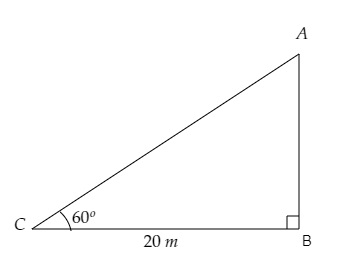# A tower stands vertically on the ground. From a point on the ground, $20 \mathrm{~m}$ away from the foot of the tower, the angle of elevation of the top of the tower is $60^{\circ}$. What is the height of the tower?

Given:

A tower stands vertically on the ground. From a point on the ground, $20 \mathrm{~m}$ away from the foot of the tower, the angle of elevation of the top of the tower is $60^{\circ}$.

To do:

We have to find the height of the tower.

Solution:Let $AB$ be the tower and $C$ is the point $20 \mathrm{~m}$ away from the foot of the tower.

From the figure,

$\mathrm{BC}=20 \mathrm{~m}, \angle \mathrm{ACB}=60^{\circ}$

Let the height of the tower be $\mathrm{AB}=h \mathrm{~m}$

We know that,

$\tan \theta=\frac{\text { Perpendicular }}{\text { Base }}$

$=\frac{\text { AB }}{BC}$

$\Rightarrow \tan 60^{\circ}=\frac{h}{20}$

$\Rightarrow \sqrt{3}=\frac{h}{20}$

$\Rightarrow h=20 \sqrt{3} \mathrm{~m}$

Therefore, the height of the tower is $20 \sqrt{3} \mathrm{~m}$.

Updated on: 10-Oct-2022

45 Views